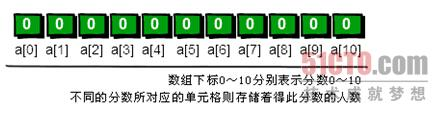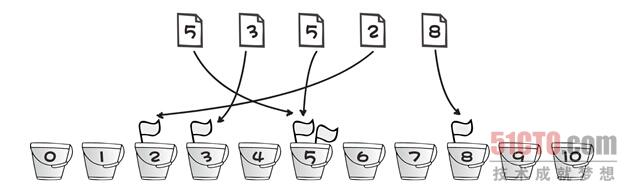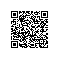# 桶排序简单入门篇^-^a为0，表示“0”没有出现过，不打印。

a为0，表示“1”没有出现过，不打印。

a为1，表示“2”出现过1次，打印2。

a为1，表示“3”出现过1次，打印3。

a为0，表示“4”没有出现过，不打印。

a为2，表示“5”出现过2次，打印5 5。

a为0，表示“6”没有出现过，不打印。

a为0，表示“7”没有出现过，不打印。

a为1，表示“8”出现过1次，打印8。

a为0，表示“9”没有出现过，不打印。

a为0，表示“10”没有出现过，不打印。

 1     #include <stdio.h>
2     int main()
3     {
4         int a,i,j,t;
5         for(i=0;i<=10;i++)
6             a[i]=0;  //初始化为0
7
8         for(i=1;i<=5;i++)  //循环读入5个数
9         {
10             scanf("%d",&t);  //把每一个数读到变量t中
11             a[t]++;  //进行计数
12         }
13
14         for(i=0;i<=10;i++)  //依次判断a~a
15             for(j=1;j<=a[i];j++)  //出现了几次就打印几次
16                 printf("%d ",i);
17
18         getchar();getchar();
19         //这里的getchar();用来暂停程序，以便查看程序输出的内容
20         //也可以用system("pause");等来代替
21         return 0;
22     } 

5 3 5 2 81     #include <stdio.h>
2
3     int main()
4     {
5         int book,i,j,t,n;
6         for(i=0;i<=1000;i++)
7             book[i]=0;
8         scanf("%d",&n);//输入一个数n，表示接下来有n个数
9         for(i=1;i<=n;i++)//循环读入n个数，并进行桶排序
10         {
11             scanf("%d",&t);  //把每一个数读到变量t中
12             book[t]++;  //进行计数，对编号为t的桶放一个小旗子
13         }
14         for(i=1000;i>=0;i--)  //依次判断编号1000~0的桶
15             for(j=1;j<=book[i];j++)  //出现了几次就将桶的编号打印几次
16                  printf("%d ",i);
17
18         getchar();getchar();
19         return 0;
20     } 

10

8 100 50 22 15 6 1 1000 999 0

1000 999 100 50 22 15 8 6 1 0

## 基数排序

### 基数排序图示• 每次分配的代价：
• 每次收集的代价：
• 总的代价为：

### 算法的c++ plus plus实现

 1 #include <iostream>
2
3 using namespace std;
4 const int MAX = 10;
5
6 void print(int *a,int sz) {
7     for(int i = 0; i < sz; i++)
8         cout << a[i] << " ";
9     cout << endl;
10 }
11
12 void RadixSortLSD(int *a, int arraySize)
13 {
14     int i, bucket[MAX], maxVal = 0, digitPosition =1 ;
15     for(i = 0; i < arraySize; i++) {
16         if(a[i] > maxVal) maxVal = a[i];
17     }
18
19     int pass = 1;  // used to show the progress
20     /* maxVal: this variable decide the while-loop count
21                if maxVal is 3 digits, then we loop through 3 times */
22     while(maxVal/digitPosition > 0) {
23         /* reset counter */
24         int digitCount = {0};
25
26         /* count pos-th digits (keys) */
27         for(i = 0; i < arraySize; i++)
28             digitCount[a[i]/digitPosition%10]++;
29
30         /* accumulated count */
31         for(i = 1; i < 10; i++)
32             digitCount[i] += digitCount[i-1];
33
34         /* To keep the order, start from back side */
35         for(i = arraySize - 1; i >= 0; i--)
36             bucket[--digitCount[a[i]/digitPosition%10]] = a[i];
37
38         /* rearrange the original array using elements in the bucket */
39         for(i = 0; i < arraySize; i++)
40             a[i] = bucket[i];
41
42         /* at this point, a array is sorted by digitPosition-th digit */
43         cout << "pass #" << pass++ << ": ";
44         print(a,arraySize);
45
46         /* move up the digit position */
47         digitPosition *= 10;
48     }
49  }
50
51 int main()
52 {
53     int a[] = {170, 45, 75, 90, 2, 24, 802, 66};
54     const size_t sz = sizeof(a)/sizeof(a);
55
56     cout << "pass #0: ";
57     print(a,sz);
59     return 0;
60 }

1 pass #0: 170 45 75 90 2 24 802 66
2 pass #1: 170 90 2 802 24 45 75 66
3 pass #2: 2 802 24 45 66 170 75 90
4 pass #3: 2 24 45 66 75 90 170 802## 桶排序（bucket sort）

### 图示### 算法的c++ plus plus描述

  1 #include <iostream>
2 #include <iomanip>
3 using namespace std;
4
5 #define NARRAY 8  /* array size */
6 #define NBUCKET 5 /* bucket size */
7 #define INTERVAL 10 /* bucket range */
8
9 struct Node
10 {
11     int data;
12     struct Node *next;
13 };
14
15 void BucketSort(int arr[]);
16 struct Node *InsertionSort(struct Node *list);
17 void print(int arr[]);
18 void printBuckets(struct Node *list);
19 int getBucketIndex(int value);
20
21 void BucketSort(int arr[])
22 {
23     int i,j;
24     struct Node **buckets;
25
26     /* allocate memory for array of pointers to the buckets */
27     buckets = (struct Node **)malloc(sizeof(struct Node*) * NBUCKET);
28
29     /* initialize pointers to the buckets */
30     for(i = 0; i < NBUCKET;++i) {
31         buckets[i] = NULL;
32     }
33
34     /* put items into the buckets */
35     for(i = 0; i < NARRAY; ++i) {
36         struct Node *current;
37         int pos = getBucketIndex(arr[i]);
38         current = (struct Node *) malloc(sizeof(struct Node));
39         current->data = arr[i];
40         current->next = buckets[pos];
41         buckets[pos] = current;
42     }
43
44     /* check what's in each bucket */
45     for(i = 0; i < NBUCKET; i++) {
46         cout << "Bucket[" << i << "] : ";
47             printBuckets(buckets[i]);
48         cout << endl;
49     }
50
51     /* sorting bucket using Insertion Sort */
52     for(i = 0; i < NBUCKET; ++i) {
53         buckets[i] = InsertionSort(buckets[i]);
54     }
55
56     /* check what's in each bucket */
57     cout << "-------------" << endl;
58     cout << "Bucktets after sorted" << endl;
59     for(i = 0; i < NBUCKET; i++) {
60         cout << "Bucket[" << i << "] : ";
61             printBuckets(buckets[i]);
62         cout << endl;
63     }
64
65     /* put items back to original array */
66     for(j =0, i = 0; i < NBUCKET; ++i) {
67         struct Node *node;
68         node = buckets[i];
69         while(node) {
70             arr[j++] = node->data;
71             node = node->next;
72         }
73     }
74
75     /* free memory */
76     for(i = 0; i < NBUCKET;++i) {
77         struct Node *node;
78         node = buckets[i];
79         while(node) {
80             struct Node *tmp;
81             tmp = node;
82             node = node->next;
83             free(tmp);
84         }
85     }
86     free(buckets);
87     return;
88 }
89
90 /* Insertion Sort */
91 struct Node *InsertionSort(struct Node *list)
92 {
93     struct Node *k,*nodeList;
94     /* need at least two items to sort */
95     if(list == 0 || list->next == 0) {
96         return list;
97     }
98
99     nodeList = list;
100     k = list->next;
101     nodeList->next = 0; /* 1st node is new list */
102     while(k != 0) {
103         struct Node *ptr;
104         /* check if insert before first */
105         if(nodeList->data > k->data)  {
106             struct Node *tmp;
107             tmp = k;
108             k = k->next;
109             tmp->next = nodeList;
110             nodeList = tmp;
111             continue;
112         }
113
114         for(ptr = nodeList; ptr->next != 0; ptr = ptr->next) {
115             if(ptr->next->data > k->data) break;
116         }
117
118         if(ptr->next!=0){
119             struct Node *tmp;
120             tmp = k;
121             k = k->next;
122             tmp->next = ptr->next;
123             ptr->next = tmp;
124             continue;
125         }
126         else{
127             ptr->next = k;
128             k = k->next;
129             ptr->next->next = 0;
130             continue;
131         }
132     }
133     return nodeList;
134 }
135
136 int getBucketIndex(int value)
137 {
138     return value/INTERVAL;
139 }
140
141 void print(int ar[])
142 {
143     int i;
144     for(i = 0; i < NARRAY; ++i) {
145         cout << setw(3) << ar[i];
146     }
147     cout << endl;
148 }
149
150 void printBuckets(struct Node *list)
151 {
152     struct Node *cur = list;
153     while(cur) {
154         cout << setw(3) << cur->data;
155         cur = cur->next;
156     }
157 }
158
159 int main(void)
160 {
161     int array[NARRAY] = {29,25,3,49,9,37,21,43};
162
163     cout << "Initial array" << endl;
164     print(array);
165     cout << "-------------" << endl;
166
167     BucketSort(array);
168     cout << "-------------" << endl;
169     cout << "Sorted array"  << endl;
170     print(array);
171     return 0;
172 }

 1 Initial array
2  29 25  3 49  9 37 21 43
3 -------------
4 Bucket :   9  3
5 Bucket :
6 Bucket :  21 25 29
7 Bucket :  37
8 Bucket :  43 49
9 -------------
10 Bucktets after sorted
11 Bucket :   3  9
12 Bucket :
13 Bucket :  21 25 29
14 Bucket :  37
15 Bucket :  43 49
16 -------------
17 Sorted array
18   3  9 21 25 29 37 43 49

## 计数排序（counting sort）

### 算法的c++ plus plus实现

 1 #include <iostream>
2
3 using namespace std;
4
5 void print(int a[], int sz) {
6     for (int i = 0; i < sz;  i++ ) cout << a[i] << " ";
7     cout << endl;
8 }
9
10 void CountingSort(int arr[], int sz) {
11     int i, j, k;
12     int idx = 0;
13     int min, max;
14
15     min = max = arr;
16     for(i = 1; i < sz; i++) {
17         min = (arr[i] < min) ? arr[i] : min;
18         max = (arr[i] > max) ? arr[i] : max;
19     }
20
21     k = max - min + 1;
22     /* creates k buckets */
23     int *B = new int [k];
24     for(i = 0; i < k; i++) B[i] = 0;
25
26     for(i = 0; i < sz; i++) B[arr[i] - min]++;
27     for(i = min; i <= max; i++)
28         for(j = 0; j < B[i - min]; j++) arr[idx++] = i;
29
30     print(arr,sz);
31
32     delete [] B;
33 }
34
35 int main()
36 {
37     int a[] = {5,9,3,9,10,9,2,4,13,10};
38     const size_t sz = sizeof(a)/sizeof(a);
39     print(a,sz);
40     cout << "----------------------\n" ;
41     CountingSort(a, sz);
42 }

1 5 9 3 9 10 9 2 4 13 10
2 ----------------------
3 2 3 4 5 9 9 9 10 10 13使用钉钉扫一扫加入圈子
+ 订阅